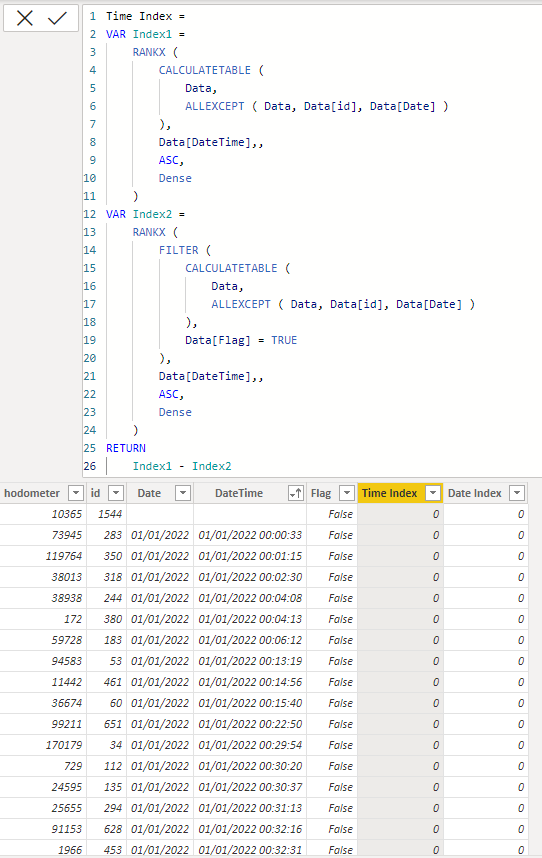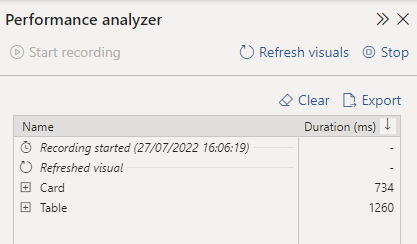cancel
Showing results for
Did you mean:Frequent Visitor

## how to summarize table with consecutive days

I need to discover water leakage by ID.
A leakage is considered when you have spent water in every hour from 0 to 6h for 5 consecutive days.
The difference of hodometer value by hour is the water spent.

hour 0, hodometer 73.95
hour 1, hodometer 74.00
difference = 0.05

For example:
A customer shows a difference > 0 in hodometer like this:
0h and 1h = 0.01

1h and 2h = 0.02

2h and 3h = 0.01

3h and 4h = 0.01

4h and 5h = 0.02

5h and 6h = 0.01

in the same day.
If this happens for 3 days in a row, it's a leak.

How to calculate this?
All approaches I tried, Power BI has gone out of memory (my laptop has 20GB RAM).
My complete dataset has 3.5Million rows.

I appreciate any help!

1 ACCEPTED SOLUTIONSuper User

Hi @bihumano
This is a bit complex. It took three calculated columns and a measure to get to the results with decent performance. Here is a sample file with the solution https://we.tl/t-2ZVnK1VClU

The criteria of 6 consecutive hours on 5 consecutive does not seem to be reasonable. I think the range need to be extended further in order to limit the number of leaks to a more reasonable value. The time difference between two consecutive readings is not exactly one hour therefore, I had to redefine it in the code as 45 - 75 min.
The code is not super fast But I guess 1.2 - 1.3 seconds for this size of data shall be acceptable.The DAX for the calculated columns:

``````Flag =
VAR CurrentDateTime =
Data[DateTime]
VAR CurrentIDDateTable =
CALCULATETABLE ( Data, ALLEXCEPT ( Data, Data[id], Data[Date] ) )
VAR FilteredTable =
FILTER ( CurrentIDDateTable, Data[DateTime] < CurrentDateTime )
VAR PreviousRecord =
TOPN ( 1, FilteredTable, Data[DateTime] )
MAXX ( PreviousRecord, Data[hodometer] )
VAR PreviousDateTime =
MAXX ( PreviousRecord, Data[DateTime] )
VAR TimeDifference =
DATEDIFF ( PreviousDateTime, CurrentDateTime, MINUTE )
VAR Check1 =
VAR Check2 =
TimeDifference < 75 && TimeDifference > 45
RETURN
Check1 && Check2``````
``````Time Index =
VAR Index1 =
RANKX (
CALCULATETABLE (
Data,
ALLEXCEPT ( Data, Data[id], Data[Date] )
),
Data[DateTime],,
ASC,
Dense
)
VAR Index2 =
RANKX (
FILTER (
CALCULATETABLE (
Data,
ALLEXCEPT ( Data, Data[id], Data[Date] )
),
Data[Flag] = TRUE
),
Data[DateTime],,
ASC,
Dense
)
RETURN
Index1 - Index2``````
``````Date Index =
VAR Index1 =
RANKX (
CALCULATETABLE (
Data,
ALLEXCEPT ( Data, Data[id] )
),
Data[Date],,
ASC,
Dense
)
VAR Index2 =
RANKX (
FILTER (
CALCULATETABLE (
Data,
ALLEXCEPT ( Data, Data[id] )
),
Data[Flag] = TRUE
),
Data[Date],,
ASC,
Dense
)
RETURN
Index1 - Index2``````

The DAX for the measure

``````Water Leaks =
VAR ConsecutiveHours = 6
VAR ConsecutiveDays = 5
RETURN
SUMX (
VALUES ( Data[id] ),
CALCULATE (
VAR T1 =
SUMMARIZE ( Data, Data[Date Index], Data[Date], Data[Time Index] )
VAR T2 =
SUMMARIZE (
T1,
Data[Date Index],
Data[Date],
"@ConsecutiveHours",
VAR CurrentDate = Data[Date]
RETURN
COUNTROWS ( FILTER ( T1, Data[Date] = CurrentDate ) )
)
VAR T3 =
FILTER ( T2, [@ConsecutiveHours] > ConsecutiveHours )
VAR T4 =
ADDCOLUMNS ( T3, "@ConsecutiveDays", COUNTROWS ( FILTER ( T3, Data[Date Index] = EARLIER ( Data[Date Index] ) ) ) )
VAR T5 =
FILTER ( T4, [@ConsecutiveDays] > ConsecutiveDays )
RETURN
IF ( NOT ISEMPTY ( T5 ), 1 )
)
)``````
3 REPLIES 3Super User

Hi @bihumano
This is a bit complex. It took three calculated columns and a measure to get to the results with decent performance. Here is a sample file with the solution https://we.tl/t-2ZVnK1VClU

The criteria of 6 consecutive hours on 5 consecutive does not seem to be reasonable. I think the range need to be extended further in order to limit the number of leaks to a more reasonable value. The time difference between two consecutive readings is not exactly one hour therefore, I had to redefine it in the code as 45 - 75 min.
The code is not super fast But I guess 1.2 - 1.3 seconds for this size of data shall be acceptable.The DAX for the calculated columns:

``````Flag =
VAR CurrentDateTime =
Data[DateTime]
VAR CurrentIDDateTable =
CALCULATETABLE ( Data, ALLEXCEPT ( Data, Data[id], Data[Date] ) )
VAR FilteredTable =
FILTER ( CurrentIDDateTable, Data[DateTime] < CurrentDateTime )
VAR PreviousRecord =
TOPN ( 1, FilteredTable, Data[DateTime] )
MAXX ( PreviousRecord, Data[hodometer] )
VAR PreviousDateTime =
MAXX ( PreviousRecord, Data[DateTime] )
VAR TimeDifference =
DATEDIFF ( PreviousDateTime, CurrentDateTime, MINUTE )
VAR Check1 =
VAR Check2 =
TimeDifference < 75 && TimeDifference > 45
RETURN
Check1 && Check2``````
``````Time Index =
VAR Index1 =
RANKX (
CALCULATETABLE (
Data,
ALLEXCEPT ( Data, Data[id], Data[Date] )
),
Data[DateTime],,
ASC,
Dense
)
VAR Index2 =
RANKX (
FILTER (
CALCULATETABLE (
Data,
ALLEXCEPT ( Data, Data[id], Data[Date] )
),
Data[Flag] = TRUE
),
Data[DateTime],,
ASC,
Dense
)
RETURN
Index1 - Index2``````
``````Date Index =
VAR Index1 =
RANKX (
CALCULATETABLE (
Data,
ALLEXCEPT ( Data, Data[id] )
),
Data[Date],,
ASC,
Dense
)
VAR Index2 =
RANKX (
FILTER (
CALCULATETABLE (
Data,
ALLEXCEPT ( Data, Data[id] )
),
Data[Flag] = TRUE
),
Data[Date],,
ASC,
Dense
)
RETURN
Index1 - Index2``````

The DAX for the measure

``````Water Leaks =
VAR ConsecutiveHours = 6
VAR ConsecutiveDays = 5
RETURN
SUMX (
VALUES ( Data[id] ),
CALCULATE (
VAR T1 =
SUMMARIZE ( Data, Data[Date Index], Data[Date], Data[Time Index] )
VAR T2 =
SUMMARIZE (
T1,
Data[Date Index],
Data[Date],
"@ConsecutiveHours",
VAR CurrentDate = Data[Date]
RETURN
COUNTROWS ( FILTER ( T1, Data[Date] = CurrentDate ) )
)
VAR T3 =
FILTER ( T2, [@ConsecutiveHours] > ConsecutiveHours )
VAR T4 =
ADDCOLUMNS ( T3, "@ConsecutiveDays", COUNTROWS ( FILTER ( T3, Data[Date Index] = EARLIER ( Data[Date Index] ) ) ) )
VAR T5 =
FILTER ( T4, [@ConsecutiveDays] > ConsecutiveDays )
RETURN
IF ( NOT ISEMPTY ( T5 ), 1 )
)
)``````Frequent VisitorSuper User

Hi @bihumano
The ID with maximum number of records has only 12 records and all in the same day. Please append some data that reflects the criteria of at least two consecutive days in order to test it properly. Thank you